Test: 1P Diode Rectifiers FW

# Test: 1P Diode Rectifiers FW

Test Description

## 20 Questions MCQ Test Power Electronics | Test: 1P Diode Rectifiers FW

Test: 1P Diode Rectifiers FW for Electrical Engineering (EE) 2023 is part of Power Electronics preparation. The Test: 1P Diode Rectifiers FW questions and answers have been prepared according to the Electrical Engineering (EE) exam syllabus.The Test: 1P Diode Rectifiers FW MCQs are made for Electrical Engineering (EE) 2023 Exam. Find important definitions, questions, notes, meanings, examples, exercises, MCQs and online tests for Test: 1P Diode Rectifiers FW below.
Solutions of Test: 1P Diode Rectifiers FW questions in English are available as part of our Power Electronics for Electrical Engineering (EE) & Test: 1P Diode Rectifiers FW solutions in Hindi for Power Electronics course. Download more important topics, notes, lectures and mock test series for Electrical Engineering (EE) Exam by signing up for free. Attempt Test: 1P Diode Rectifiers FW | 20 questions in 20 minutes | Mock test for Electrical Engineering (EE) preparation | Free important questions MCQ to study Power Electronics for Electrical Engineering (EE) Exam | Download free PDF with solutions
 1 Crore+ students have signed up on EduRev. Have you?
Test: 1P Diode Rectifiers FW - Question 1

### A single-phase full wave mid-point type diode rectifier requires __________ number of diodes whereas bridge type requires _________

Detailed Solution for Test: 1P Diode Rectifiers FW - Question 1

A bridge type requires 4 diodes which are connected in a bridge, and the mid-point has 2 diodes.

Test: 1P Diode Rectifiers FW - Question 2

### A single-phase full wave rectifier is a

Detailed Solution for Test: 1P Diode Rectifiers FW - Question 2

It is a two-pulse rectifier as it generates 2 pulses per cycle.

Test: 1P Diode Rectifiers FW - Question 3

### The below shown circuit is that of a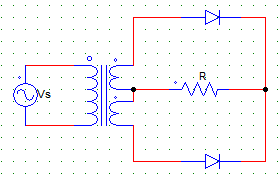Detailed Solution for Test: 1P Diode Rectifiers FW - Question 3

Full wave M-2 type employs a transformer and two diodes.

Test: 1P Diode Rectifiers FW - Question 4

A single-phase full wave rectifier is a

Detailed Solution for Test: 1P Diode Rectifiers FW - Question 4

It is a two-pulse rectifier as it generates 2 pulses per cycle.

Test: 1P Diode Rectifiers FW - Question 5

In a 1-phase full wave bridge rectifier with M-2 type of connection has secondary side voltage Vs = Vm sin ωt, with R load & ideal diodes.The expression for the average value of the output voltage can be given by

Detailed Solution for Test: 1P Diode Rectifiers FW - Question 5

The voltage waveform is a pulsating voltage with peak value Vm & symmetrical about π.
Vo = (1/π) ∫π Vm sinωt d(ωt)

Test: 1P Diode Rectifiers FW - Question 6

The below shown circuit is that of a​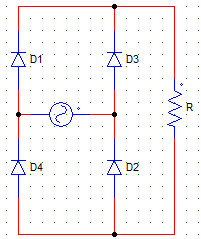Detailed Solution for Test: 1P Diode Rectifiers FW - Question 6

Full wave B-2 type uses 4 diodes in a bridge connection.

Test: 1P Diode Rectifiers FW - Question 7

For the circuit shown below, find the power delivered to the R load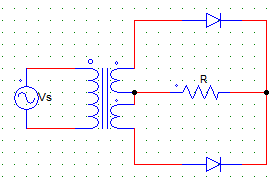Where,
Vs = 230V
Vs is the secondary side single winding rms voltage.
R = 1KΩ

Detailed Solution for Test: 1P Diode Rectifiers FW - Question 7

Power delivered to the load is V(rms).I(rms) = V(rms)2/R
Where, for the given circuit, V(rms) = Vs.

Test: 1P Diode Rectifiers FW - Question 8

The PIV experienced by the diodes in the mid-point type configuration is

Detailed Solution for Test: 1P Diode Rectifiers FW - Question 8

In the m-2 type connection, each diode experiences a reverse voltage of 2Vm.

Test: 1P Diode Rectifiers FW - Question 9

For the circuit shown below, find the value of the average output current.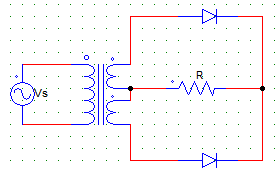Where,
Vs = 230V
R = 1KΩ
Vs is the secondary side single winding rms voltage.

Detailed Solution for Test: 1P Diode Rectifiers FW - Question 9

I = Vo/R
Vo = 2Vm/π.

Test: 1P Diode Rectifiers FW - Question 10

In the circuit, let Im be the peak value of the sinusoidal source current. The average value of the diode current for the below given configuration is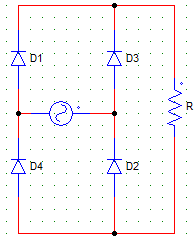Detailed Solution for Test: 1P Diode Rectifiers FW - Question 10

The peak current through the diodes is same as that passing from the load. Each diode pair conducts for 180 degrees. Hence, 1/2 of a cycle. Average current = Im/2.

Test: 1P Diode Rectifiers FW - Question 11

The PIV experienced by each of the diodes for the below shown rectifier configuration is​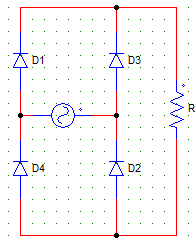Detailed Solution for Test: 1P Diode Rectifiers FW - Question 11

When any diode is reversed biased due to the negative half cycle, the maximum peak value through it will be Vm.

Test: 1P Diode Rectifiers FW - Question 12

For the circuit shown in the figure below,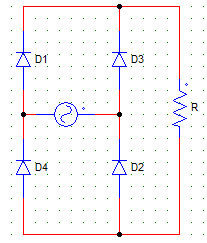Vs = 230 V
R = 10Ω
Find the average value of output current.

Detailed Solution for Test: 1P Diode Rectifiers FW - Question 12

I = Vo/R
Vo = 2Vm/π.

Test: 1P Diode Rectifiers FW - Question 13

Choose the correct statement regarding the below given circuit.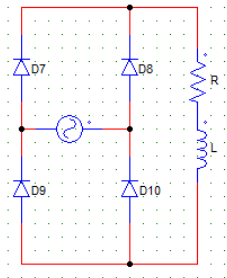Detailed Solution for Test: 1P Diode Rectifiers FW - Question 13

Due to the inductive nature of the load, the Diodes are force conducted & voltage goes negative but the current can never fall below zero.

Test: 1P Diode Rectifiers FW - Question 14

For a single phase, full bridge, diode rectifier excited from a 230 V, 50 Hz source. With R = 10 Ω & the inductance(L) large enough to maintain continues conduction, the average and rms values of diode currents will be

Detailed Solution for Test: 1P Diode Rectifiers FW - Question 14

Id(avg) = Io/2 = Vo/2R
Id(rms) = Io/√2 = Vo/R√2.

Test: 1P Diode Rectifiers FW - Question 15

The circuit shown below, will have the output voltage waveform similar to that of a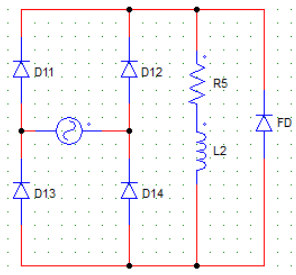Detailed Solution for Test: 1P Diode Rectifiers FW - Question 15

The FD short circuits the load & voltage waveform is similar to that of a Full wave bridge rectifier with R load.

Test: 1P Diode Rectifiers FW - Question 16

For the circuit shown below, the load current attains the maximum value at ωt =​Detailed Solution for Test: 1P Diode Rectifiers FW - Question 16

As the load is RL, the load current will be maximum when the output voltage waveform falls to zero i.e. at π. At π the inductor is charged to its maximum value and starts delivering power to the source.

Test: 1P Diode Rectifiers FW - Question 17

For a single phase, full bridge, diode rectifier excited from a 230 V, 50 Hz source. With R = 10 Ω & the inductance(L) large enough to maintain continuous conduction, the value of the supply power factor will be

Detailed Solution for Test: 1P Diode Rectifiers FW - Question 17

Pf = Vs.Is.cosθ/Vo.Io
Io = Vo/R A
Vo = 2Vm/π Volts.

Test: 1P Diode Rectifiers FW - Question 18

The rectification efficiency for B-2 type & M-2 type full wave diode rectifiers are ___ & ___ respectively.

Detailed Solution for Test: 1P Diode Rectifiers FW - Question 18

B-2 type has efficiency 8/π. M-2 type has efficiency half of that of a B-2 type.

Test: 1P Diode Rectifiers FW - Question 19

A load of R = 60 Ω is fed from 1phase, 230 V, 50 Hz supply through a step-up transformer & than a diode. The transformer turns ratio = 2. The power delivered to the load is

Detailed Solution for Test: 1P Diode Rectifiers FW - Question 19

P = Vo2/R
Vo = Vm/π
AC supplied to the rectifier is 2 x 230 = 460 V (rms)
Therefore, Vo = √2 x 460 / π = 207.04
P = 714.43 W.

Test: 1P Diode Rectifiers FW - Question 20

For the circuit shown below, D11 & D14 conduct from?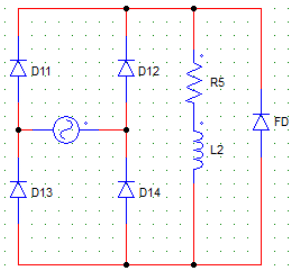Assume that anode of D12 is positive at ωt = 0 and likewise.

Detailed Solution for Test: 1P Diode Rectifiers FW - Question 20

In the first cycle i.e. 0 to π, D12 and D13 conduct. In the next cycle i.e. π to 2π, D11 and D14 conduct.

## Power Electronics

5 videos|39 docs|63 tests
Information about Test: 1P Diode Rectifiers FW Page
In this test you can find the Exam questions for Test: 1P Diode Rectifiers FW solved & explained in the simplest way possible. Besides giving Questions and answers for Test: 1P Diode Rectifiers FW, EduRev gives you an ample number of Online tests for practice

## Power Electronics

5 videos|39 docs|63 tests(Scan QR code)